# Dijkstra’s shortest path with minimum edges

Prerequisite: Dijkstra’s shortest path algorithm
Given an adjacency matrix graph representing paths between the nodes in the given graph. The task is to find the shortest path with minimum edges i.e. if there a multiple short paths with same cost then choose the one with the minimum number of edges.

Consider the graph given below: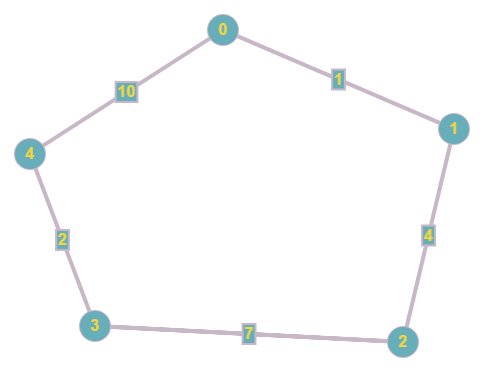There are two paths from vertex 0 to vertex 3 with the weight of 12:

1. 0 -> 1 -> 2 -> 3
2. 0 -> 4 -> 3

Since, Dijkstra’s algorithm is a greedy algorithm that seeks the minimum weighted vertex on every iteration, so the original Dijkstra’s algorithm will output the first path but the result should be the second path as it contains minimum number of edges.

Examples:

Input: graph[][] = { {0, 1, INFINITY, INFINITY, 10},
{1, 0, 4, INFINITY, INFINITY},
{INFINITY, 4, 0, 7, INFINITY},
{INFINITY, INFINITY, 7, 0, 2},
{10, INFINITY, INFINITY, 2, 0} };
Output: 0->4->3
INFINITY here shows that u and v are not neighbors

Input: graph[][] = { {0, 5, INFINITY, INFINITY},
{5, 0, 5, 10},
{INFINITY, 5, 0, 5},
{INFINITY, 10, 5, 0} };
Output: 0->1->3

## Recommended: Please try your approach on {IDE} first, before moving on to the solution.

Approach: The idea of the algorithm is to use the original Dijkstra’s algorithm, but also to keep track on the length of the paths by an array that stores the length of the paths from the source vertex, so if we find a shorter path with the same weight, then we will take it.

Let’s follow the upper example iteration by iteration:
Consider we want to find the shortest path from vertex 0 to vertex 3

Initial state: The distance and the parent of all vertices are Infinity and NILL respectively, as usual.
But now, we have one more array called pathlength[] that stores the length of the path from the source vertex to all of the vertices.
Initially, we set all the elements of pathlength[] to 0.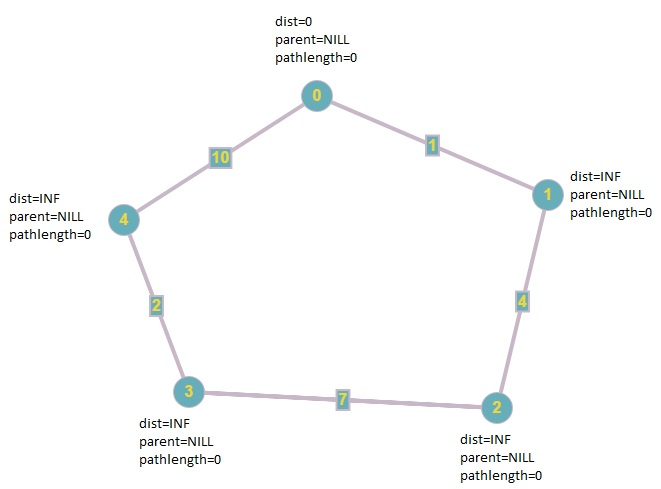First iteration: First we seek the vertex which contains the minimum distance which is vertex 0, as shown in the image above.
Then, we traverse all its neighbors which are not blackened, which are 1 and 4. Since, the distance of vertex 1 and 4 are infinity, then we reduce their weights to 1 and 10 respectively. Update the parents, and set the pathlength[] for each vertex (1 and 4) to 1 because they can be reached from source vertex by 1 edge.
After that, we blacken the vertex as the original Dijkstra’s algorithm does.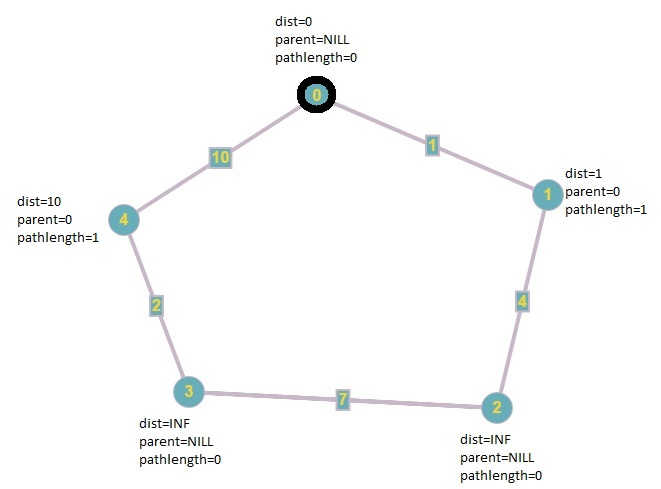Second iteration: We continue to seek the non-blakened vertex which contains the minimum distance, which is vertex 1, and after that we reduce its neighbor’s weight to 1 + 4 = 5 and update its parent as the original Dijkstra’s algorithm does, and set its pathlength[] to 2, because it is two edges away from the source vertex.
Finally, we blacken vertex 1.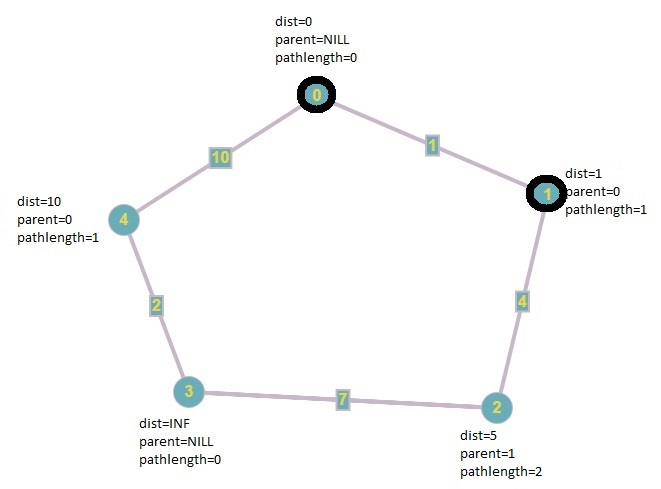Third iteration: Again, the non-blackened vertex which contains the minimum distance is vertex 2, so we update the non-blackened neighbors. It has one non-blackened neighbor which is vertex 3. So, we update its weight from Infinity to 5 + 7 = 12 then we set its parent to 2 and set its pathlength[] to 3 because it is 3 edges sway from the source vertex.
Finally, we blacken vertex 2.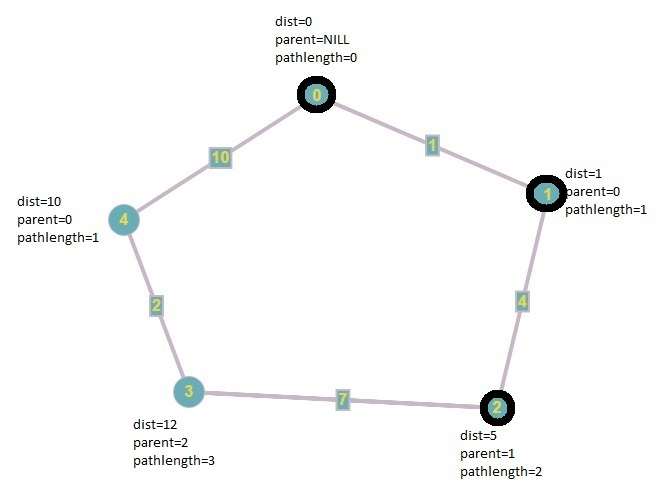Fourth iteration: In this iteration, the algorithm acts different from the original Dijkstra’s algorithm. We seek the non-blackened vertex which contains the minimum distance which is 4. Since, the distance to vertex 3 from the source vertex is 12 (0->1->2->3) and the distance of vertex 4 plus the edge (4, 3) is 12, it means that we just found a new path to vertex 3 from the source vertex with the same weight. Then, we check if the new path is shorter (in edges) than the existing one and we take the one with the minimum edges.
Finally, we blacken vertex 4.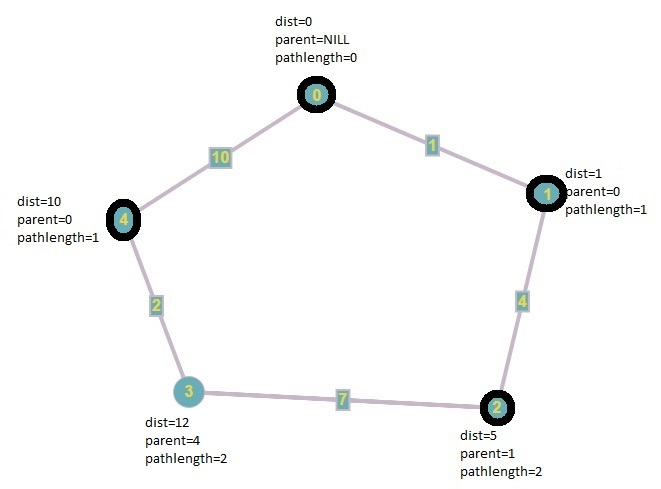Since V-1 vertices are blackened, the algorithm ends.

Below is the implementation of the above approach:

## C++

 `// C++ program to find the shortest path ` `// with minimum edges in a graph ` `#include ` `using` `namespace` `std; ` `#define INFINITY 9999 ` `#define n 5 ` `#define s 0 ` `#define d 3 ` `#define NILL -1 ` `int` `MinDistance(``int``*, ``int``*); ` `void` `PrintPath(``int``*, ``int``); ` ` `  `// Function to find the shortest path ` `// with minimum edges in a graph ` `void` `Dijkstra(``int` `Graph[n][n], ``int` `_n, ``int` `_s, ``int` `_d) ` `{ ` ` `  `    ``int` `i, u, v, count; ` `    ``int` `dist[n]; ` `    ``int` `Blackened[n] = { 0 }; ` `    ``int` `pathlength[n] = { 0 }; ` `    ``int` `parent[n]; ` ` `  `    ``// The parent Of the source vertex is always equal to nill ` `    ``parent[_s] = NILL; ` ` `  `    ``// first, we initialize all distances to infinity. ` `    ``for` `(i = 0; i < n; i++) ` `        ``dist[i] = INFINITY; ` ` `  `    ``dist[_s] = 0; ` `    ``for` `(count = 0; count < n - 1; count++) { ` `        ``u = MinDistance(dist, Blackened); ` ` `  `        ``// if MinDistance() returns INFINITY, then the graph is not ` `        ``// connected and we have traversed all of the vertices in the ` `        ``// connected component of the source vertex, so it can reduce ` `        ``// the time complexity sometimes ` `        ``// In a directed graph, it means that the source vertex ` `        ``// is not a root ` `        ``if` `(u == INFINITY) ` `            ``break``; ` `        ``else` `{ ` ` `  `            ``// Mark the vertex as Blackened ` `            ``Blackened[u] = 1; ` `            ``for` `(v = 0; v < n; v++) { ` `                ``if` `(!Blackened[v] && Graph[u][v] ` `                    ``&& dist[u] + Graph[u][v] < dist[v]) { ` `                    ``parent[v] = u; ` `                    ``pathlength[v] = pathlength[parent[v]] + 1; ` `                    ``dist[v] = dist[u] + Graph[u][v]; ` `                ``} ` `                ``else` `if` `(!Blackened[v] && Graph[u][v] ` `                         ``&& dist[u] + Graph[u][v] == dist[v] ` `                         ``&& pathlength[u] + 1 < pathlength[v]) { ` `                    ``parent[v] = u; ` `                    ``pathlength[v] = pathlength[u] + 1; ` `                ``} ` `            ``} ` `        ``} ` `    ``} ` ` `  `    ``// Printing the path ` `    ``if` `(dist[_d] != INFINITY) ` `        ``PrintPath(parent, _d); ` `    ``else` `        ``cout << ``"There is no path between vertex "` `             ``<< _s << ``"to vertex "` `<< _d; ` `} ` ` `  `int` `MinDistance(``int``* dist, ``int``* Blackened) ` `{ ` `    ``int` `min = INFINITY, min_index, v; ` `    ``for` `(v = 0; v < n; v++) ` `        ``if` `(!Blackened[v] && dist[v] < min) { ` `            ``min = dist[v]; ` `            ``min_index = v; ` `        ``} ` `    ``return` `min == INFINITY ? INFINITY : min_index; ` `} ` ` `  `// Function to print the path ` `void` `PrintPath(``int``* parent, ``int` `_d) ` `{ ` `    ``if` `(parent[_d] == NILL) { ` `        ``cout << _d; ` `        ``return``; ` `    ``} ` `    ``PrintPath(parent, parent[_d]); ` `    ``cout << ``"->"` `<< _d; ` `} ` ` `  `// Driver code ` `int` `main() ` `{ ` `    ``// INFINITY means that u and v are not neighbors. ` `    ``int` `Graph[n][n] = { { 0, 1, INFINITY, INFINITY, 10 }, ` `                        ``{ 1, 0, 4, INFINITY, INFINITY }, ` `                        ``{ INFINITY, 4, 0, 7, INFINITY }, ` `                        ``{ INFINITY, INFINITY, 7, 0, 2 }, ` `                        ``{ 10, INFINITY, INFINITY, 2, 0 } }; ` `    ``Dijkstra(Graph, n, s, d); ` `    ``return` `0; ` `} `

## Java

 `// Java program to find the shortest path  ` `// with minimum edges in a graph  ` `import` `java.io.*; ` `import` `java.util.*; ` ` `  `class` `GFG  ` `{ ` ` `  `    ``static` `int` `INFINITY = ``9999``, n = ``5``, s = ``0``, d = ``3``, NILL = -``1``; ` ` `  `    ``// Function to find the shortest path ` `    ``// with minimum edges in a graph ` `    ``static` `void` `Dijkstra(``int``[][] Graph, ``int` `_n, ``int` `_s, ``int` `_d)  ` `    ``{ ` ` `  `        ``int` `i, u, v, count; ` `        ``int``[] dist = ``new` `int``[n]; ` `        ``int``[] Blackened = ``new` `int``[n]; ` `        ``int``[] pathlength = ``new` `int``[n]; ` `        ``int``[] parent = ``new` `int``[n]; ` ` `  `        ``// The parent Of the source vertex is always equal to nill ` `        ``parent[_s] = NILL; ` ` `  `        ``// first, we initialize all distances to infinity. ` `        ``for` `(i = ``0``; i < n; i++) ` `            ``dist[i] = INFINITY; ` ` `  `        ``dist[_s] = ``0``; ` `        ``for` `(count = ``0``; count < n - ``1``; count++)  ` `        ``{ ` `            ``u = MinDistance(dist, Blackened); ` ` `  `            ``// if MinDistance() returns INFINITY, then the graph is not ` `            ``// connected and we have traversed all of the vertices in the ` `            ``// connected component of the source vertex, so it can reduce ` `            ``// the time complexity sometimes ` `            ``// In a directed graph, it means that the source vertex ` `            ``// is not a root ` `            ``if` `(u == INFINITY) ` `                ``break``; ` `            ``else` `            ``{ ` ` `  `                ``// Mark the vertex as Blackened ` `                ``Blackened[u] = ``1``; ` `                ``for` `(v = ``0``; v < n; v++)  ` `                ``{ ` `                    ``if` `(Blackened[v] == ``0` `&& Graph[u][v] != ``0`  `                        ``&& dist[u] + Graph[u][v] < dist[v])  ` `                    ``{ ` `                        ``parent[v] = u; ` `                        ``pathlength[v] = pathlength[parent[v]] + ``1``; ` `                        ``dist[v] = dist[u] + Graph[u][v]; ` `                    ``}  ` `                    ``else` `if` `(Blackened[v] == ``0` `&& Graph[u][v] != ``0`  `                            ``&& dist[u] + Graph[u][v] == dist[v] ` `                            ``&& pathlength[u] + ``1` `< pathlength[v]) ` `                    ``{ ` `                        ``parent[v] = u; ` `                        ``pathlength[v] = pathlength[u] + ``1``; ` `                    ``} ` `                ``} ` `            ``} ` `        ``} ` ` `  `        ``// Printing the path ` `        ``if` `(dist[_d] != INFINITY) ` `            ``PrintPath(parent, _d); ` `        ``else` `            ``System.out.println(``"There is not path between vertex "` `+ ` `                                ``_s + ``" to vertex "` `+ _d); ` `    ``} ` ` `  `    ``static` `int` `MinDistance(``int``[] dist, ``int``[] Blackened) ` `    ``{ ` `        ``int` `min = INFINITY, min_index = -``1``, v; ` `        ``for` `(v = ``0``; v < n; v++) ` `            ``if` `(Blackened[v] == ``0` `&& dist[v] < min) ` `            ``{ ` `                ``min = dist[v]; ` `                ``min_index = v; ` `            ``} ` `        ``return` `min == INFINITY ? INFINITY : min_index; ` `    ``} ` ` `  `    ``// Function to print the path ` `    ``static` `void` `PrintPath(``int``[] parent, ``int` `_d) ` `    ``{ ` `        ``if` `(parent[_d] == NILL) ` `        ``{ ` `            ``System.out.print(_d); ` `            ``return``; ` `        ``} ` `        ``PrintPath(parent, parent[_d]); ` `        ``System.out.print(``"->"` `+ _d); ` `    ``} ` ` `  `    ``// Driver Code ` `    ``public` `static` `void` `main(String[] args) ` `    ``{ ` ` `  `        ``// INFINITY means that u and v are not neighbors. ` `        ``int``[][] Graph = { { ``0``, ``1``, INFINITY, INFINITY, ``10` `}, ` `                        ``{ ``1``, ``0``, ``4``, INFINITY, INFINITY }, ` `                        ``{ INFINITY, ``4``, ``0``, ``7``, INFINITY }, ` `                        ``{ INFINITY, INFINITY, ``7``, ``0``, ``2` `}, ` `                        ``{ ``10``, INFINITY, INFINITY, ``2``, ``0` `} }; ` `        ``Dijkstra(Graph, n, s, d); ` `    ``} ` `} ` ` `  `// This code is contributed by ` `// sanjeev2552 `

## Python

 `# Python program to find the shortest path ` `# with minimum edges in a graph ` `def` `Dijkstra(Graph, _s, _d): ` `    ``row ``=` `len``(Graph) ` `    ``col ``=` `len``(Graph[``0``]) ` `    ``dist ``=` `[``float``(``"Inf"``)] ``*` `row ` `    ``Blackened ``=``[``0``] ``*` `row ` `    ``pathlength ``=``[``0``] ``*` `row ` `    ``parent ``=` `[``-``1``] ``*` `row ` `    ``dist[_s]``=` `0` `    ``for` `count ``in` `range``(row``-``1``): ` `        ``u ``=` `MinDistance(dist, Blackened) ` ` `  `        ``# if MinDistance() returns INFINITY, then the graph is not ` `        ``# connected and we have traversed all of the vertices in the ` `        ``# connected component of the source vertex, so it can reduce ` `        ``# the time complexity sometimes ` `        ``# In a directed graph, it means that the source vertex ` `        ``# is not a root ` `        ``if` `u ``=``=` `float``(``"Inf"``): ` `            ``break` `        ``else``: ` ` `  `            ``# Mark the vertex as Blackened ` `            ``Blackened[u]``=` `1`  `        ``for` `v ``in` `range``(row): ` `            ``if` `Blackened[v]``=``=` `0` `and` `Graph[u][v] ``and` `dist[u]``+``Graph[u][v]"``, _d, ` `def` `MinDistance(dist, Blackened): ` `    ``min` `=` `float``(``"Inf"``) ` `    ``for` `v ``in` `range``(``len``(dist)): ` `        ``if` `not` `Blackened[v] ``and` `dist[v]<``min``: ` `            ``min` `=` `dist[v] ` `            ``Min_index ``=` `v ` `    ``return` `float``(``"Inf"``) ``if` `min` `=``=` `float``(``"Inf"``) ``else` `Min_index ` ` `  `# Driver code ` `# float("Inf") means that u and v are not neighbors ` `Graph ``=``[[``0``, ``1``, ``float``(``"Inf"``), ``float``(``"Inf"``), ``10``], ` `       ``[``1``, ``0``, ``4``, ``float``(``"Inf"``), ``float``(``"Inf"``)], ` `       ``[``float``(``"Inf"``), ``4``, ``0``, ``7``, ``float``(``"Inf"``)], ` `       ``[``float``(``"Inf"``), ``float``(``"Inf"``), ``7``, ``0``, ``2``], ` `       ``[``10``, ``float``(``"Inf"``), ``float``(``"Inf"``), ``2``, ``0``]] ` `Dijkstra(Graph, ``0``, ``3``) `

Output:

```0->4->3
```

GeeksforGeeks has prepared a complete interview preparation course with premium videos, theory, practice problems, TA support and many more features. Please refer Placement 100 for details

My Personal Notes arrow_drop_upCheck out this Author's contributed articles.

If you like GeeksforGeeks and would like to contribute, you can also write an article using contribute.geeksforgeeks.org or mail your article to contribute@geeksforgeeks.org. See your article appearing on the GeeksforGeeks main page and help other Geeks.

Please Improve this article if you find anything incorrect by clicking on the "Improve Article" button below.

Improved By : sanjeev2552